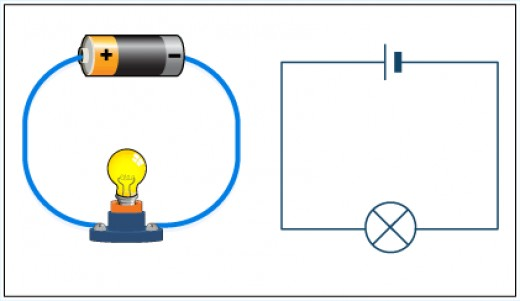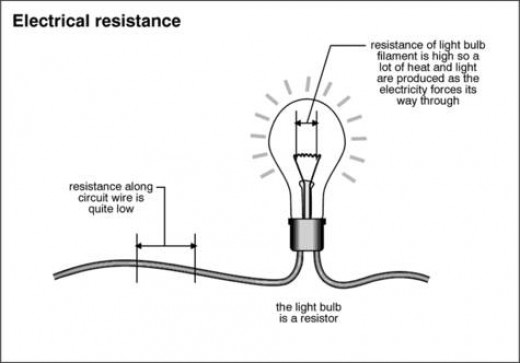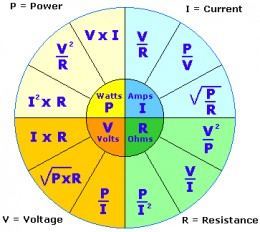# Watts, Amps and Volts and How To Understand ElectricityWatt is the Amp and Volt? Simple Electrical Circuit with a Bulb, some Wire and a Battery

## So What Are Watts, Amps and Volts All About?

A guide to the basics of electricity. Here is everything you need to know about electrical power (Watts), current (Amps) and voltage (Volts), how to understand simple electricity and the way these parameters relate to one another.

Lets begin with some simple definitions:

## Amps

Electricity consists of the flow of electrons through a conductor such as an electric wire. You can’t see electrons but a helpful analogy is to think of electricity as the flow of water through a pipe.

We measure the rate of flow of electricity as an electric current (just as we think of the rate of flow of water in a river as the river current). The letter used to represent current in an equation is I.

Electric current is measured in Amperes, shortened to Amps or simply the letter A.

A current of 2 Amps can be written as 2A. The bigger the current the more electricity is flowing.

## Volts

So what makes the current flow in the first place? A device such as a battery provides what is known as a potential difference in an electric circuit.

If we go back to our water analogy, the battery is like a water pump that propels water through a pipe.

It creates pressure in the pipe causing the water to flow. So how do we measure this pressure? We call electrical pressure Voltage and measure it in Volts, shortened to V. The letter V is also used represent Voltage in an equation.

A voltage of 3 volts can be written as 3V.

The bigger the voltage the higher the pressure and the more current flows. But in order for the current to flow, the electrical conductor or wire must loop back to the battery.

If we break the circuit, with a switch for example, then no current will flow.

## Resistance

Before we get onto Power and Watts we need to understand Resistance. The letter R is used to represent resistance in an equation.

In the case of our battery, if we short circuited it with the wire (no bulb), the wire and battery would get very hot and the battery would soon be flat.

That is because there would be virtually no resistance in the circuit so a huge electrical current would flow until the battery was empty.

But once we add our bulb to the circuit, the bulb offers a lot of resistance and creates a local ‘blockage’ (or narrowing of the pipe) where the current finds it hard to flow.

This greatly reduces the current flowing in the circuit which means the energy in the battery is released more slowly.

As the battery forces the current through the bulb the battery’s energy is released in the bulb in the form of light (and heat).

In other words, the current carries stored energy from the battery to the bulb where it is turned into light and heat energy.

Resistance is measured in Ohms or Ω (Omega) for short.

So 5 Ohms can be written 5Ω.Watt is the Amp and Volt? Electrical ResistanceWatt are Amps and Volts?

## Watts

So how do Current and Voltage relate to one another? Well, the bigger the current the brighter the light and similarly the bigger the voltage the brighter the light.

Both the voltage and the current in the bulb determine how much energy is released in a certain time.

The Watt is a measure of power or how much energy is released per second. It can be shortened to W.

1 Watt can be written 1W.

We can calculate the power released in a bulb by multiplying the voltage in Volts across the bulb by the current in Amps flowing through the bulb (W = V x I).

For example a current of 2 Amps flowing through a bulb with 12 Volts across it generates 24 watts of power.

In the UK, domestic power is supplied at 240 Volts. A 100 Watt bulb will therefore draw a current of 100/240 Amps (about 0.4A). This means a 1A fuse can be safely added to the mains plug of a desk lamp with a 100W bulb because 1A is much greater than 0.4A.

In the USA, the domestic supply is typically 110V, safer than the UK, and this means a 100W bulb draws a current of 100/110 Amps (about 0.9A).## Simple Calculations

These simple calculations are summarised in the formulae in the circle to the right. So, for example, we can calculate the power in Watts (see orange area top left of the circle) as V x I.

Hopefully now you now understand the difference between electric current, measured in Amps, voltage, measured in Volts or electrical power measured in Watts.

Finally, remember that if you know the voltage of your power supply then you can calculate the current through a bulb, fuse or other electrical appliance or component, based on its rating in Watts.